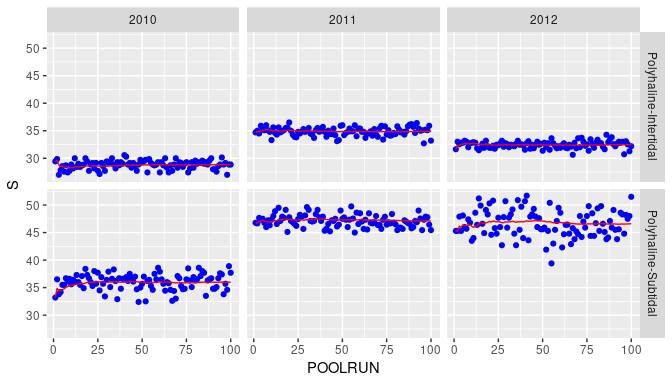Introduction to the benthos-package

Introduction

The benthos-package provides functions for analysing benthic data sets. To use the benthos package, some basic knowledge of programming in R (R Core Team, 2017; www.r-project.org) is assumed.

The functions in the benthos-package have been designed to integrate seamlessly with those of the dplyr-package (Wickham et al., 2017). The dplyr-package implements a grammar of data manipulation to make data analysis more efficient and clear.

The benthos-package is designed to use the forward-pipe operator (%>%) provided by the magrittr-package (Milton Bache & Wickham, 2014). This operator can be used for chaining multiple data operations together. This reduces the need for temporary variables or nested function calls and leads to cleaner and more readable code. Consult the references below and the corresponding package vignettes for more information.

The benthos-package follows the same philosophy as the dplyr package: in stead of providing complicated functions that can do many tasks (‘Swiss army knife’-functions), we provide a set of ‘small functions that each do one thing well’ (Wickham & Francois, 2017; ‘Introduction to dplyr vignette’). As a consequence, you will not find functions in this package which perform a complete analysis, rather it provides basic building blocks that you can use to build your own functions and applications.

The benthos-package can be attached by means of

library(benthos)

In this vignette, we also attach the dplyr, tidyr, readr and ggplot2 packages for data manipulation and visualization.

library(dplyr)
library(tidyr)
library(ggplot2)

Sample data set

In the sections below, we will illustrate the benthos-package by means of the Oosterschelde marine benthos data set. This data set ships with the benthos-package and can be loaded by typing:

data(oosterschelde)

The first 10 records of these data set are given below:

oosterschelde
# A tibble: 4,269 x 8
OBJECTID     SAMPLEID DATE          ID HABITAT               AREA TAXON                COUNT
<chr>           <int> <date>     <int> <chr>                <dbl> <chr>                <int>
1 nl89_oostsde    14956 2010-09-06     1 Polyhaline-Subtidal 0.0157 Aphelochaeta marioni     1
2 nl89_oostsde    14956 2010-09-06     1 Polyhaline-Subtidal 0.0157 Crangon crangon          1
3 nl89_oostsde    14956 2010-09-06     1 Polyhaline-Subtidal 0.0157 Nephtys hombergii        4
4 nl89_oostsde    14956 2010-09-06     1 Polyhaline-Subtidal 0.0157 Oligochaeta              5
5 nl89_oostsde    14956 2010-09-06     1 Polyhaline-Subtidal 0.0157 Pygospio elegans        12
6 nl89_oostsde    14956 2010-09-06     1 Polyhaline-Subtidal 0.0157 Scoloplos armiger        1
7 nl89_oostsde    14956 2010-09-06     1 Polyhaline-Subtidal 0.0157 Spio martinensis         1
8 nl89_oostsde    14956 2010-09-06     1 Polyhaline-Subtidal 0.0157 Spiophanes bombyx        8
9 nl89_oostsde    14957 2010-09-06     2 Polyhaline-Subtidal 0.0157 Corophium arenarium      1
10 nl89_oostsde    14957 2010-09-06     2 Polyhaline-Subtidal 0.0157 Nephtys cirrosa          1
# … with 4,259 more rows

Type

?oosterschelde

to see the documentation of this data set.

Preprocessing

Data preprocessing is an important first step. In this section we will demonstrate some preprocessing steps.

Selecting variables and observations

As a simple preprocessing step, we will only consider samples (stored in rows) taken in August and September. These can be selected as follows:

Standardization of taxon names

Taxon names need to be standardized to comply with the names in the WoRMS-database. The as_accepted-function does this conversion by using the TWN-list (http://sofus.ecosys.nl/taxabase.htm). This list is based on the WoRMS database (World Register of Marine Species, http://www.marinespecies.org).

We can use the is_accepted-function to check if a taxon complies with WoRMS:

This returns a logical vector with TRUE if a taxon complies with WoRMS and FALSE otherwise. The total number of records, compliant names, and missing names is given below

Taxa not compliant with WoRMS (if any) are given below and will be removed:

oosterschelde %>%
filter(!is_accepted(taxon = TAXON))
# A tibble: 19 x 8
OBJECTID     SAMPLEID DATE          ID HABITAT                 AREA TAXON     COUNT
<chr>           <int> <date>     <int> <chr>                  <dbl> <chr>     <int>
1 nl89_oostsde    14964 2010-09-06     8 Polyhaline-Subtidal   0.0774 Crustacea     1
2 nl89_oostsde    14972 2010-09-06    16 Polyhaline-Subtidal   0.0157 Insecta       1
3 nl89_oostsde    14983 2010-09-07    17 Polyhaline-Subtidal   0.0157 Insecta       1
4 nl89_oostsde    15005 2010-09-07    39 Polyhaline-Subtidal   0.0157 Crustacea     1
5 nl89_oostsde    15025 2010-09-10    59 Polyhaline-Intertidal 0.0157 Insecta       3
6 nl89_oostsde    15075 2010-09-24    73 Polyhaline-Intertidal 0.0157 Insecta       1
7 nl89_oostsde    15468 2011-08-18   134 Polyhaline-Intertidal 0.0157 Animalia      1
8 nl89_oostsde    15260 2011-09-08   199 Polyhaline-Subtidal   0.0157 Animalia      1
9 nl89_oostsde    15507 2011-09-13   205 Polyhaline-Intertidal 0.0157 Animalia      1
10 nl89_oostsde    15241 2011-09-13   206 Polyhaline-Intertidal 0.0157 Animalia      1
11 nl89_oostsde    15509 2011-09-14   215 Polyhaline-Intertidal 0.0157 Animalia      1
12 nl89_oostsde    15631 2012-08-29   295 Polyhaline-Subtidal   0.0774 Animalia      1
13 nl89_oostsde    15633 2012-08-27   297 Polyhaline-Subtidal   0.0157 Crustacea     1
14 nl89_oostsde    15651 2012-08-29   315 Polyhaline-Subtidal   0.0157 Animalia      1
15 nl89_oostsde    15675 2012-08-27   339 Polyhaline-Subtidal   0.0157 Animalia      1
16 nl89_oostsde    15707 2012-09-18   369 Polyhaline-Intertidal 0.0157 Animalia      1
17 nl89_oostsde    15708 2012-09-06   370 Polyhaline-Intertidal 0.0157 Animalia      1
18 nl89_oostsde    15710 2012-08-20   372 Polyhaline-Intertidal 0.0157 Animalia      1
19 nl89_oostsde    15720 2012-08-20   382 Polyhaline-Intertidal 0.0157 Animalia      1
oosterschelde <- oosterschelde %>%
filter(is_accepted(taxon = TAXON))

Other examples of the usage of the is_accepted and as_accepted-functions are:

If we want to make sure that all taxa names comply with WoRMS we simply use:

Taxon names that are not in the WoRMS/TWN-list get name NA (not available):

In our case all names comply with those in WoRMS.

Genus to species conversion

Genus to species conversion reallocates the counts of taxa that are identified at the genus level to taxa in the same sampling unit and of the same genus but that are identified on the species level. The redistribution of counts is proportional to the number of counts of taxa at the species level (Van Loon et al., 2015).

The Oosterschelde data set only contains individuals at the genus and species level (individuals at higher order taxonomic levels have been removed for didactic purposes only).

It is convenient to split each taxon into its generic and specific name. This can be accomplished as follows:

Both functions generic_name and specific_name return NA if TAXON is not a valid binomial. That is the case if a taxon has only been identified on the genus level. For example:

We will create a new column to indicate these cases.

The number of taxa that has been identified at the genus level is

Genus to species conversion is performed for each genus in a sample by means of the genus_to_species function: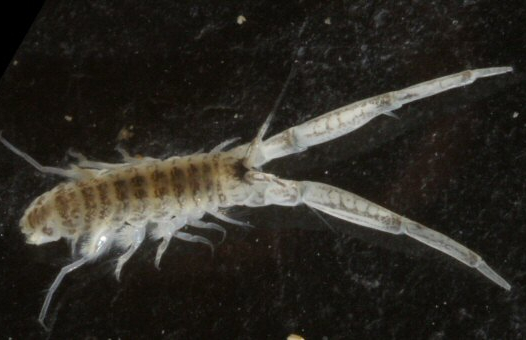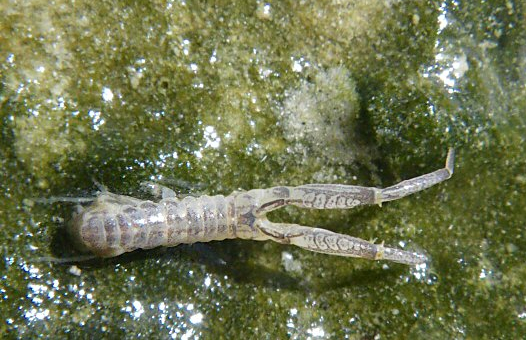Corophium arenarium (left) and Corophium volutator (right) (source: http://nature22.com)

To illustrate the algorithm, consider all records with generic name Corophium in sample 130:

In this sample, the genus Corophium is identified 19 times at the species level, i.e., 17 times as Corophium arenarium and twice as Corophium volutator. For five individuals, the analyst was unable to identify the species name, and only reported the genus. The genus to species algorithm now proportionally reallocates the $$5$$ individuals at the genus level to the taxa at the species level. That is, an additional $$5 \times \frac{17}{17 + 2} = 4.47$$ individuals will be classified as Corophium arenarium and $$5 \times \frac{2}{17 + 2} = 0.53$$ as Corophium volutator.

Note that the total number of species will not be affected:

To finalize our analysis, we will set COUNT to the value of NEWCOUNT, and remove redundant columns and records.

Data pooling

Analysis results make only sense when all sampling units are collected on the same support. That is not the case for the oosterschelde data:

We distinguish three different supports (0.0157, 0.0236, 0.0774 m2). In this section, we will demonstrate how to pool these data to approximately the same support in the range from 0.09 to 0.11 m2. See Van Loon et al. (2015) for more details.

We will only pool samples of the same year, so we’ll start by adding a new column to our table containing the year:

Next, we will randomly pool samples for each HABITAT and YEAR. We will pool several times (in the example below n_pool_runs = 10), to reduce the effect of pool composition and to make sure that each sample will be represented in a pool (no leftovers on average).

This procedure will return al list of pool identifiers (POOLID) for each pool run:

Each row in this table corresponds to the row with the same index in oosterschelde. Therefore, it is quite easy to combine this table with the ‘oosterschelde’ data:

It was not always possible to use all samples for pooling. These ‘leftover’ samples have POOLID NA. However, on average each sample has been pooled between 5 and 10 times.

For further analysis, it is convenient to convert this table from ‘wide’ to ‘long’-format. This can be done efficiently by functions of the tidyr-package (Wickham, 2017), which provides an interesting framework to tidy your data (Wickham, 2014).

To check if the pooling algorithm succeeded in its task, we compute the area of each pool. These areas should vary between 0.09 and 0.11 m2, i.e., our target area.

The following code computes the frequencies of the available areas:

This is also visualized in the bar graph below. All the pooled areas are nicely within the target area demarcated by the red lines.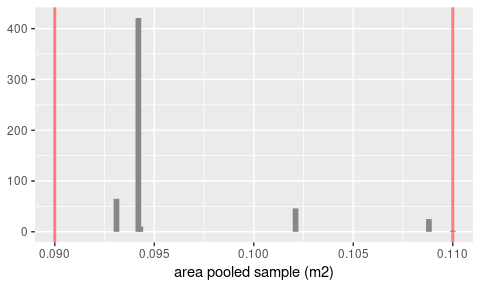The pooled samples can be used to estimate biodiversity measures (see the next section for details). For instance, the species richness for each pool is given by:

The annual mean species richness for each habitat and year is given by:

Biodiversity measures

Several biodiversity measures have been implemented in the benthos-package. In the sections below, we will demonstrate how to calculate these measures. To simplify things, all analysis will be performed on a single sampling unit:

d <- oosterschelde %>%
filter(HABITAT == "Polyhaline-Subtidal", YEAR == 2010, POOLRUN == 1, POOLID == 1) %>%
select(TAXON, COUNT) %>%
arrange(TAXON)
d
# A tibble: 41 x 2
TAXON                  COUNT
<chr>                  <dbl>
1 Angulus tenuis             1
2 Aphelochaeta marioni       1
3 Aphelochaeta marioni      25
4 Bathyporeia                2
5 Capitella capitata         2
6 Cossura longocirrata       1
7 Echinocardium cordatum     1
8 Ensis                      1
9 Lanice conchilega          4
10 Magelona johnstoni         2
# … with 31 more rows

Measures of species abundance

Total abundance

The total abundance is the total number of individuals in a sampling unit, and is computed by:

Measures of species richness

Species richness

Species richness $$S$$ is the number of different species in a (pooled) sample. It can be computed by means of

Margalef’s index of diversity

Species richness $$S$$ is strongly dependent on sampling size. Margalef’s diversity index $$D_\mathrm{M}$$ takes sampling size into account. It is given by $D_\mathrm{M} = \frac{S-1}{\ln(N)}$ where $$N$$ is the total abundance, i.e, the total number of individuals in the sampling unit. In case $$N=1$$, this index will be set to zero.

It can be computed for a specific sampling unit by:

Rygg’s index of diversity

Species richness $$S$$ is strongly dependent on sampling size. Like Margalef’s diversity index $$D_\mathrm{M}$$, Rygg’s index of diversity takes sampling size into account (Rygg, 2006). It is given by $SN = \frac{\ln{S}}{\ln(\ln(N))}$ where $$N$$ is the total abundance, i.e, the total number of individuals in the sampling unit.

It can be computed for a specific sampling unit by:

Rygg’s index shows some inconsistencies for small N and S ((S=2, N=2), (S=2, N=3) and (S=3, N=3)). This is illustrated in the third figure below. As a reference, also Margalef’s index is given in the top figure.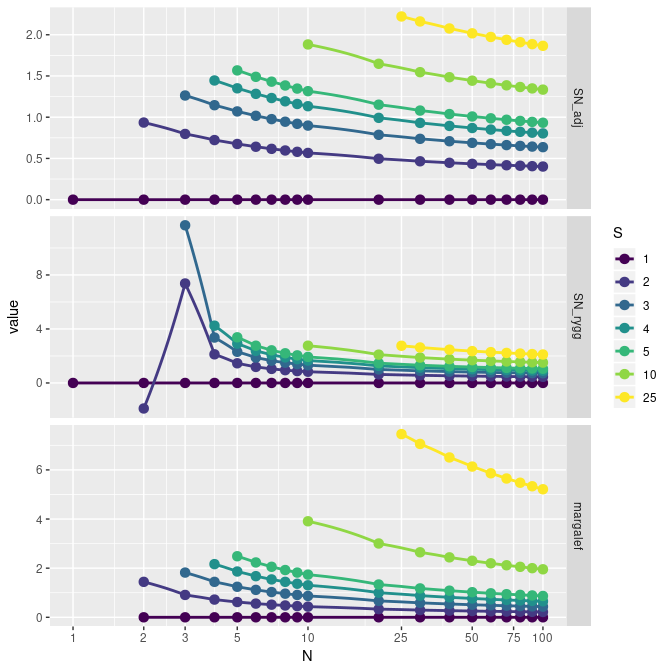The second figure shows a graph based on the adjusted version of Rygg’s index. It is given by:

$SNA = \frac{\ln{S}}{\ln(\ln(N+1)+1)}$

The adjusted version of Rygg’s index can be computed by means of:

Hurlbert’s $$\mathrm{E}(S_n)$$

Hurlbert (1971) gives the expected number of species in a sample of n individuals selected at random (without replacement) from a collection of N individuals and S species:

$\mathrm{E}(S_n) = \sum_{i=1}^S \left[1 - \frac{\binom{N-N_i}{n}}{\binom{N}{n}} \right]$

Contrary to species richness, this measure is not dependent on the number of individuals. It can be computed for a specific sampling unit by:

$$\mathrm{E}(S_n)$$ can be computed for $$n \in {1, 2, \dots, N}$$, where $$N$$ is the total abundance. This has been done in the figure below.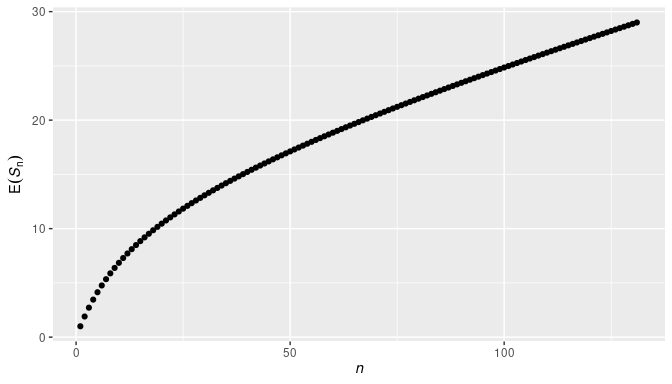Note that $$\mathrm{E}(S_n)$$ can be computed for $$n \leq N$$. Extrapolation, i.e. $$n > N$$, is not possible.

Measures of heterogeneity/evenness

Simpson’s Measure of Concentration

Simpson’s Measure of Concentration gives the probability that two individuals selected at random from a sample will belong to the same species. For an infinite sample Simpson’s Index is given by: $\lambda = \sum_{i=1}^S \pi_i^2$ where $$\pi_i$$ the proportion of the individuals in species $$i$$. For a finite sample, Simpson’s index is: $L = \sum_{i=1}^S \frac{n_i (n_i-1)}{N (N-1)}$ where $$n_i$$ the number of individuals in species $$i$$ and $$N$$ the total number of individuals.

The finite sample case can be computed by:

Hurlbert’s Probability of Interspecific Encounter (PIE)

Related to Simpson’s index is Hurlbert’s probability of inter-specific encounter (PIE). It gives the probability that two individuals selected at random (without replacement) from a sample will belong to different species (Hurlbert, 1971, p.579, Eq. 3): $\Delta_1 = \sum_{i=1}^S \left(\frac{N_i}{N}\right)\left(\frac{N-N_i}{N-1}\right) = \left(\frac{N}{N-1}\right)\Delta_2$ where $$\Delta_2$$ (Hurlbert, 1971, p.579, Eq. 4) is the probability that two individuals selected at random (with replacement) from a sample will belong to different species: $\Delta_2 = 1 - \sum_{i=1}^S \pi_i^2$ where $$N_i$$ is the number of individuals of the $$i$$th species in the community, $$N$$ is the total number of individuals in the community, $$\pi_i = N_i/N$$, and $$S$$ is the number of species in the community.

Hurlbert’s PIE can be computed by means of:

Note that it is the complement of Simpson’s Measure of Concentration (for finite sample sizes):

Shannon’s Index

Shannon’s index (or entropy) is given by:

$H' = -\sum_i p_i \log_2 p_i$ where $$p_i$$ is the proportion of individuals found in taxon $$i$$. It can be computed for a specific sampling unit by:

Hill’s Diversity Numbers

According to Hill (1973): ‘a diversity number is figuratively a measure of how many species are present if we examine the sample down to a certain depth among its rarities. If we examine superficially (e.g., by using $$N_2$$) we shall see only the more abundant species. If we look deeply e.g. by using $$N_0$$ we shall see all the species present.’. His diversity number is given by: $N_a = \left(\sum_{i=1}^S p_i^a\right)^{1/(1-a)}$

Depending on parameter $$a$$, Hill’s numbers gradually give more weight to the rarest species (small $$a$$) or most common species (large $$a$$).

Special cases are:

• $$N_0$$: total number of species present
• $$N_1$$: $$\exp(H')$$, where $$H'$$: Shannon’s index
• $$N_2$$: reciprocal of Simpson’s index ($$\frac{1}{\lambda}$$)

or (efficient) short cuts:

The figure below shows Hill’s Diversity Number as function of $$a$$. From right to left, the focus is more and more on rare species.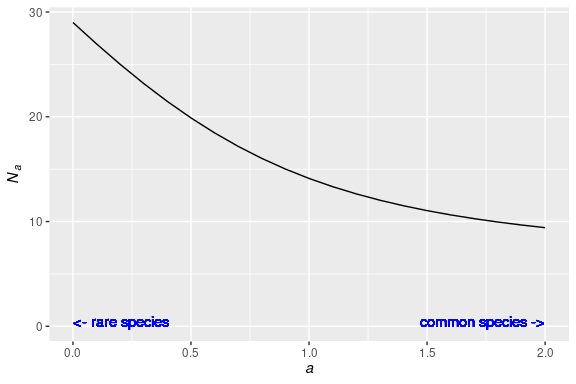Measures of species sensitivity

AZTI Marine Biotic Index (AMBI)

Borja et al. (2000) introduced the Biotic Coefficient. The expression in their paper can be rewritten as: $c_\mathrm{b} = \frac{3}{2} \sum_{i=2}^5 (i-1) p_i$ where $$\mathrm{p}$$ is a vector of length 5 containing the proportions of species in the sensitivity classes (I, II, III, IV, V) respectively.

It can be computed for a specific sampling unit by:

The accuracy of the AMBI depends (among other things) on the number of taxa for which a sensitivity group is available. The has_ambi function indicates if a group has been assigned to a taxa or not: Taxa with an AMBI sensitivity group are

The percentage of the total abundance without an AMBI group is given below

Infaunal Trophic Index (ITI)

The infaunal trophic index (ITI) is calculated as: $\mathrm{ITI} = 100 \sum_{i=1}^3 \frac{(4-i)}{3} p_i$ where $$p_i$$ is the proportion of species in class $$i$$, where

• class 1 are suspension feeders (highest quality);
• class 2 are interface feeders;
• class 3 are surface deposit feeders and
• class 4 are subsurface deposit feeders (lowest quality).

We can estimate the ITI by means of:

The accuracy of the ITI depends (among other things) on the number of taxa for which a sensitivity group is available. The has_iti function indicates if a group has been assigned to a taxa or not: Taxa with an ITI sensitivity group are

The percentage of the total abundance without an ITI group is given below

In the previous section we used $$10$$ pool runs. But is this sufficient to stabilize the results? In this section we will demonstrate the effect of pooling on the average species richness. We will reuse large sections of the code given earlier in this document: